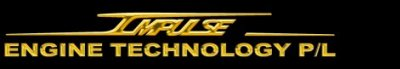Home Performance Kits Crate Engines New Products How it Works Installers Distributors Contact Us History FAQ Links
 ﻿
Negative pressure produces the SAME force as positive pressure

Negative pressure (vacuum) and positive pressure (boost) produce the SAME FORCE...the only difference is that negative pressure pulls inward and positive pressure pushes outward

While it�s understood that negative pressure (vacuum) can not be reduced below zero, the force of a vacuum is not understood as well as the force produced by positive pressure (boost). Also, there is a false perception that only 14.7psi of negative pressure (vacuum) exists between 14.7psi at sea level and zero pressure.

The fact is there�s billions of psi of negative pressure (vacuum) between 14.7psi and zero pressure but negative pressure (vacuum) is measured in air molecules per cc instead of psi. For example, the best man-made vacuums contain less than 100,000 air molecules per cc compared to about 30 billion billion (30�1018) air molecules per cc at sea level. The tremendous force produced by the best man-made vacuums is equivalent to the force produced by 440 thousand billion psi of positive pressure.

Therefore, the force of the vacuum in a typical engine can be increased a lot more than 14.7psi shown on the vacuum gauge.

However, measuring vacuum in air molecules per cc makes it difficult to compare the force of a vacuum to the same force produced by psi of positive pressure. Therefore, the example below uses psi of negative pressure to demonstrate that whether the same pressure differential is below or above 14.7psi at sea level it produces the SAME force.

For example,

If the pressure outside the engine is +15psi measured at sea level then...
 • Increasing the pressure outside the engine to +38psi produces a pressure differential of 23psi • Reducing the pressure inside the engine to -8psi also produces the SAME pressure differential of 23psi and therefore the SAME force as increasing the pressure above sea level

Reference
Vacuum is measured in air molecules - The Columbia Electronic Encyclopedia, Sixth Edition.

 Impulse Engine Technology P/L PO Box 986 Randwick NSW 2031 Australia Ph: (02) 9398 5544 Fax: (02) 9398 5644 International: Ph: +(612) 9398 5544 Fax: +(612) 9398 5644 Email Us Inventor of Negative Pressure Supercharging